# Least Pth-Norm Optimal FIR Filter Design

This example shows how to design least Pth-norm FIR filters with the `firlpnorm` function. This function uses a least-Pth unconstrained optimization algorithm to design FIR filters with arbitrary magnitude response.

### FIRLPNORM Syntax

The syntax for `firlpnorm` is similar to that of `iirlpnorm` (see the Least Pth-Norm Optimal IIR Filter Design example for details) except that the denominator order is not specified.

The function designs optimal FIR filters in the least-Pth sense. However the filter is not constrained to have linear-phase, that is, the impulse response has no special symmetry properties.

However, the linear-phase constraint also results in filters with larger order than the more general nonlinear-phase designs. Note that in some hardware implementations, one can reduce the number of multipliers in half when implementing linear-phase filters because of the symmetry in the coefficients. For example, consider the following `firlpnorm` design

```N = 30; F = [0 0.3 0.45 1]; E = F; A = [1 1 0 0]; W = [1 1 10 10]; b = firlpnorm(N,F,E,A,W); fvtool(b,1);```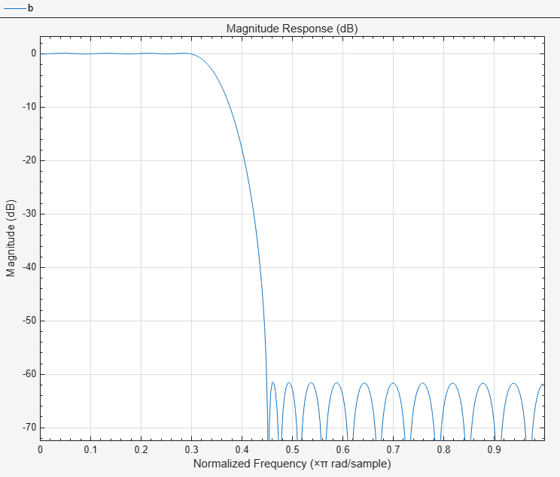If we zoom in, we can see that the filter has a passband peak ripple of about 0.008 and a stopband peak ripple of about 0.000832. A `firpm` or `firgr` design with comparable specs will require a 37th order filter. This is especially significant considering that `firgr` will provide the lowest order linear-phase FIR filter that meets the specifications.

```dev = [0.008 0.000832]; bgr = firgr('minorder',F,A,dev); orderfirgr = length(b)-1; fprintf('Order: %d\n',orderfirgr);```
```Order: 30 ```
```h = fvtool(b,1,bgr,1); legend(h,'FIRLPNORM design','FIRGR design');```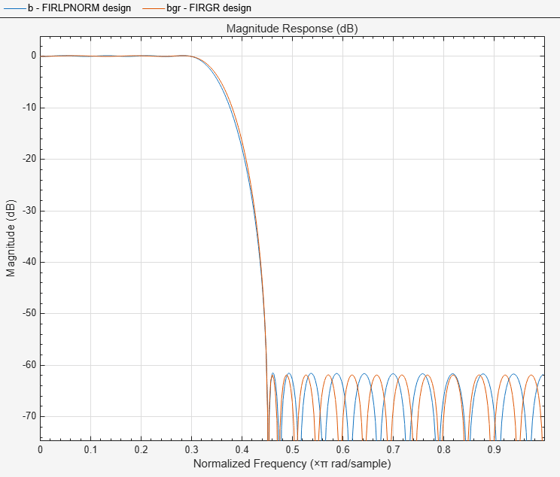Another way to look at this is by using the `firceqrip` function which also designs linear-phase equiripple filters, but whose specifications are given in a different way to `firgr` (see the constrained equiripple FIR filter design example for details). If we want a linear-phase filter of 30th order that meets the passband and stopband ripple that the design from `firlpnorm` achieves we need to live with a larger transition width.

```bceq = firceqrip(30,(F(2)+F(3))/2,dev); h = fvtool(b,1,bceq,1); legend(h,'FIRLPNORM design','FIRCEQRIP design');```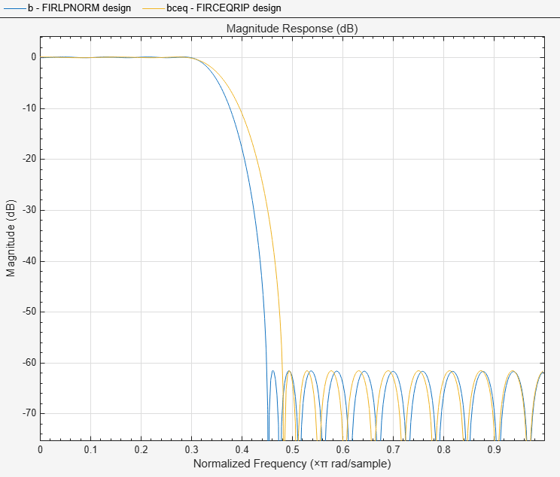### Minimum-Phase Designs

Of course it is also possible to design nonlinear-phase filters with `firgr` by specifying the `minphase` option. Doing so allows us to obtain an FIR filter of lower order than in the linear-phase case and still meet the required specs. However, even in this case, the result is a non-optimal nonlinear-phase filter because the filter order is larger than the minimum required for a nonlinear-phase equiripple filter to meet the specs as is evident from the following example.

```bm = firgr('minorder',F,A,dev,'minphase'); orderfirgrmin = length(bm)-1; fprintf('Order: %d\n',orderfirgrmin);```
```Order: 32 ```
```h = fvtool(b,1,bm,1); legend(h,'FIRLPNORM design','FIRGR minimum-phase design');```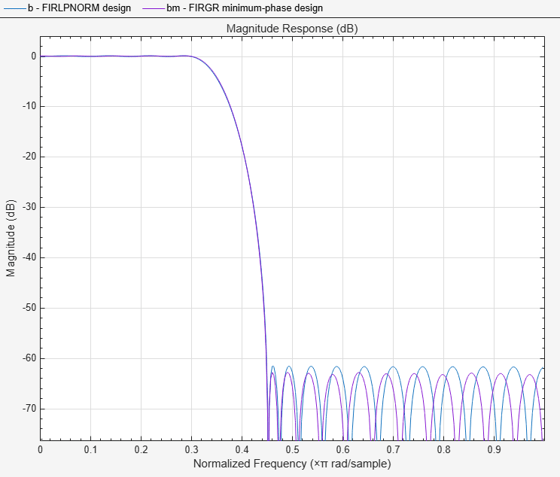### Minimum-Phase Designs with FIRLPNORM

`firlpnorm` does allow for the option to constrain the zeros to lie on or inside the unit circle, resulting in a minimum-phase design. The constraint, however, results in larger passband ripple and less stopband attenuation than the unconstrained design.

```bmlp = firlpnorm(30,F,E,A,W,'minphase'); h = fvtool(b,1,bmlp,1); legend(h,'FIRLPNORM design','FIRLPNORM minimum-phase design');```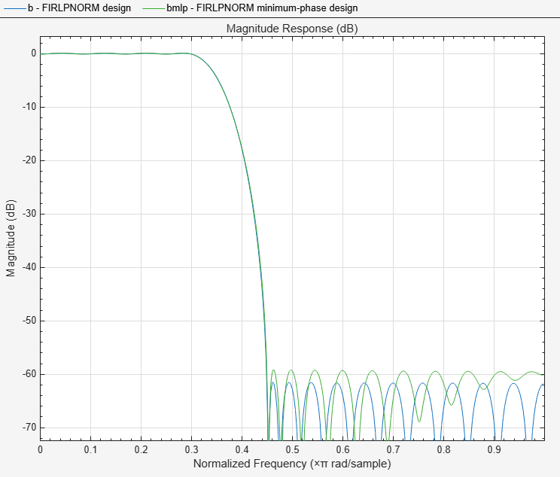If we increase the order to that of the minimum-phase filter designed with `firgr` we can see that we meet the specs met by both the 30th order `firlpnorm` (nonminimum-phase) design and the 32nd order `firgr` minimum-phase design.

```bmlp = firlpnorm(orderfirgrmin,F,E,A,W,'minphase'); h = fvtool(b,1,bm,1,bmlp,1); legend(h,'FIRLPNORM design',... 'FIRGR minimum-phase design',... 'FIRLPNORM minimum-phase design');```### Changing the Pth-Norm

Like `iirlpnorm` and `iirlpnormc`, `firlpnorm` allows for the specification of the Pth-norm used to optimize the filter. The Pth-norm is specified in the exact same way as in `iirlpnorm`, i.e. a two element vector with Pinit and Pfinal as its elements. Pinit specifies the initial Pth-norm used by the algorithm (this aids in the convergence) and Pfinal specifies the final Pth-norm with which the filter is optimized.

For example, a least-squares design for the above specs can be obtained as follows:

```N = 40; F = [0 0.4 0.45 1]; E = F; A = [0 0 1 1]; W = [1 1 10 10]; P = [2 2]; bl2 = firlpnorm(N,F,E,A,W,P); h = fvtool(bl2,1); legend(h,'FIRLPNORM design')```### Comparing to FIRLS

In comparison, we design a linear-phase least-squares filter using `firls`. Once again, for the same filter order, the linear-phase constraint results in less stopband attenuation and a larger passband ripple.

```W = [1 20]; bls = firls(N,F,A,W); h = fvtool(bl2,1,bls,1); legend(h,'FIRLPNORM design','FIRLS design');```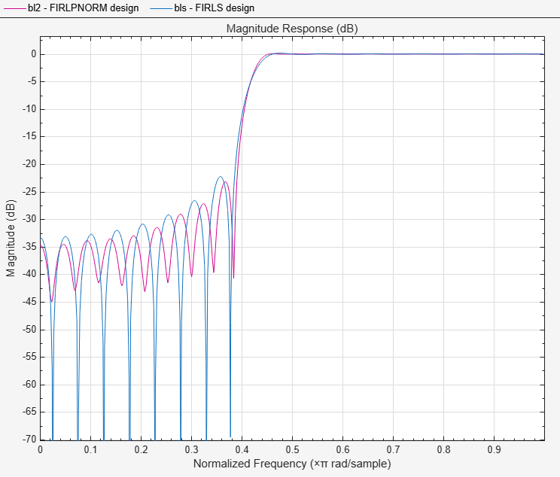### Other Norms

Equiripple designs are useful when one requires the smallest possible order to meet a set of design specifications. To meet the same specs with a least-squares design requires a higher order filter. However, the higher order does provide extra attenuation (less ripple) for a large portion of the stopband (passband). In fact least-squares design minimize the energy of the stopband. Compromises between equiripple design and least-squares design can be reached by using intermediate norms. For example we show the design of a filter with the same specs, but optimized for the following norms: 2, 4, 12, 256 (approx. infinity norm).

```W = [1 1 10 10]; P4 = [2 4]; bl4 = firlpnorm(N,F,E,A,W,P4); P12 = [2 12]; bl12 = firlpnorm(N,F,E,A,W,P12); Pinf = [2 256]; blinf = firlpnorm(N,F,E,A,W,Pinf); h = fvtool(bl2,1,bl4,1,bl12,1,blinf,1); legend(h,'P = 2','P = 4','P = 12','P = 256');```In order to meet the minimum stopband attenuation of the equiripple (256-norm) case, it is necessary to increase the order of the other designs.